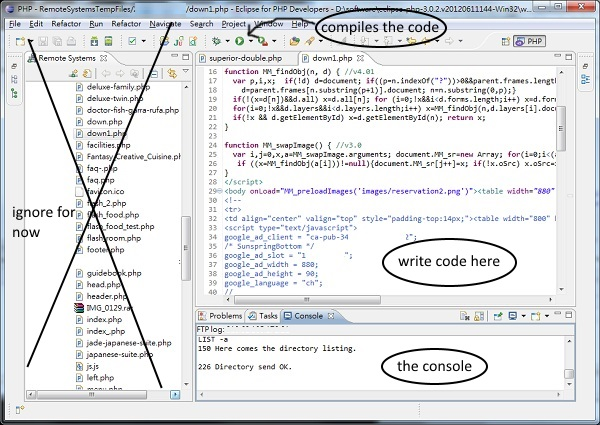# Java: Absolute Basics

A lot of sites often immediately drop you into a deluge of obscure key words and scary terms such as "Inheritance" and "Object-Oriented." I definitely didn't appreciate that when I was first learning how to code. To be fair, it can be difficult to differentiate between an absolute beginner and someone trying Java for the first time. This guide is meant for people who are just brand new to how programming works.

The following guide will hopefully describe and demonstrate all the most basic terms of Java without too much hassle.

# Part 1: Java interface in a few sentences

## The IDE

This will explain the programs you use while programming.

The IDE is an application on a computer in which you write and run code. An online IDE you can use is Ideone (limited for advanced use, but saves the hassle of downloading stuff).

A compiler is something that you use to turn the code that you write into a format that the computer can read and carry out. To compile code you have to press a button, usually labeled "compile."

The console is a text area in which errors in your code or other messages of your choice will be displayed.The above image shows the different parts of an IDE (this one is Eclipse).

# Part 2: Comments, Variables and math operations

## Comments

Comments are bits of text in code that don't carry out any functions. They are mainly used to describe to a user what parts of a code do. They are denoted in code by two forward slashes "//" and are often green in IDEs. I'll be using them frequently to give descriptions in code.

```//This is a comment. It doesn't count as code, and is ignored by a compiler.
complicatedMethod(a, b, c, d);  //This code is super complicated.
```

## Variables

Variables are the fundamental units of programming. Operators are things you do with variables to modify them, like add/subtract or assign them values.

## `int` keyword

Programming variables are kind of like algebra variables. Take a look at the following piece of code:

```int x = 10;
```

When you first learned algebra, you probably had to write out what each variable value was. "Let x equal 5, let y equal 12, etc." That's similar to what we're doing here.

`int` means that we're declaring a new integer variable. The following letter x is the name of the variable. Basically, we're creating a new variable named x that can hold an integer. In this case, x is set to 10. This is because of the `=` symbol, which is an operator which assigns a variable to a specific value. So this entire line of code is saying "A variable named x is being assigned the integer value of 10." Or in short, x gets 10.

Once you declare a new variable with int, you don't have to redeclare it again. To demonstrate:

```int x = 10;
x = 5;
```

x is first set to the value 10. Afterwards, we see that x is set to the value 5, but since it was already declared as an integer with `int` in the first line we don't have to do that again in the second line. You can also reassign values to variables. Although x was set to 10 in the first line, in the second line this value was changed to 5. Any code after that would assume that x = 5, not x = 10.

The name of a variable doesn't have to be just one letter, either. It can be a lot of different things, as long as they don't begin with a number. Here are some examples.

```int x = 2;                  //remember, comments don't affect code.
int x2 = 12;                //numbers can be in names as long as they are not first (like 2x).
int numberOfPotatoes = 12;  //names can be long...
int AAAA = 456;             //all caps too if you want
int 1 = 3;                  //this DOESN'T WORK! You can't use only a number for a variable name.
int y = 5;                  //this works
x = 12;                     //and you can always change the value of a variable like this.
```

The "=" operator assigns a variable a certain value.

## Math Operations

You can do lots of math things with variables, as you might assume. Here's a quick list of them. Assume that x and y are assigned certain integer values.

```int q = x + y;      //q is set to the value of x + y (x plus y).
q = x * y;          //r is set to the value of x * y (x times y).
q = x - y;          //s is set to the value of x - y (x minus y).
q = x / y;          //t is set to the value of x / y (x divided by y).
q = x % y;          //u is set to the remainder of x / y.
q = Math.pow(x, y);     //v is set to x^y (x to the y power).
```

The `%` operator (called the mod operator) can be a bit confusing, so I'll give an example.

```int remainder = 25 % 7;      //remainder is set to 4.
```

Why is variable `remainder` set to 4? Because 25 divided by 7 gives a remainder of 4.